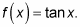##### Pre-Calculus For DummiesThe tangent function has a parent graph just like any other function. Using the graph of this function, you can make the same type of transformation that applies to the parent graph of any function. The easiest way to remember how to graph the tangent function is to remember that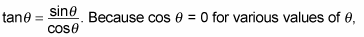some interesting things happen to tangent's graph. When the denominator of a fraction is 0, the fraction is undefined. Therefore, the graph of tangent has asymptotes, which is where the function is undefined, at each of these places.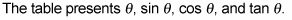It shows the roots (or zeros), the asymptotes (where the function is undefined), and the behavior of the graph in between certain key points on the unit circle.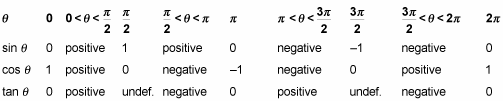To plot the parent graph of a tangent function f(x) = tan x where x represents the angle in radians, you start out by finding the vertical asymptotes. Those asymptotes give you some structure from which you can fill in the missing points.

1. Find the vertical asymptotes so you can find the domain.

These steps use x instead of theta because the graph is on the x-y plane. In order to find the domain of the tangent function f(x) = tan x, you have to locate the vertical asymptotes. The first asymptote occurs when the angle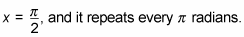(Note: The period of the tangent graph is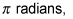which is different from that of sine and cosine.) Tangent, in other words, has asymptotes when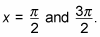The easiest way to write this is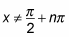where n is an integer. You write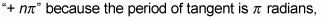so if an asymptote is at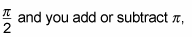you automatically find the next asymptote.

2. Determine values for the range.

Recall that the tangent function can be defined as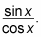The closer you get to the values where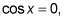the smaller the number on the bottom of the fraction gets and the larger the value of the overall fraction gets — in either the positive or negative direction.

The range of tangent has no restrictions; you aren't stuck between 1 and –1, like with sine and cosine. In fact, the ratios are any and all numbers. The range is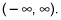3. Calculate the graph's x-intercepts.

Tangent's parent graph has roots (it crosses the x-axis) at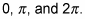You can find these values by setting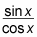equal to 0 and then solving. The x-intercepts for the parent graph of tangent are located wherever the sine value is 0.

4. Figure out what's happening to the graph between the intercepts and the asymptotes.

• The graph of f(x) = tan x is positive for angles in the first quadrant (with respect to the unit circle) and points upward toward the asymptote at pi/2, because all sine and cosine values are positive for angles in the first quadrant.

• The graph of f(x) = tan x is negative for angles in Quadrant II because sine is positive and cosine is negative for angles in this quadrant.

• The graph of f(x) = tan x is positive for angles in Quadrant III because both sine and cosine are negative.

• Finally, the graph of f(x) = tan x is positive for angles in Quadrant IV because sine is negative and cosine is positive for angles in this quadrant.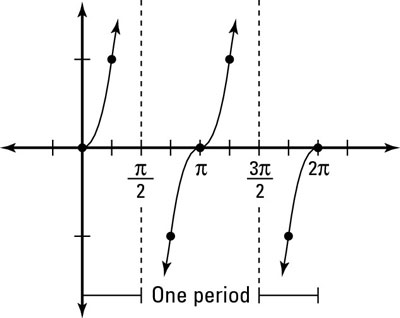Note: A tangent graph has no maximum or minimum points.

The figure shows the parent graph of tangent,